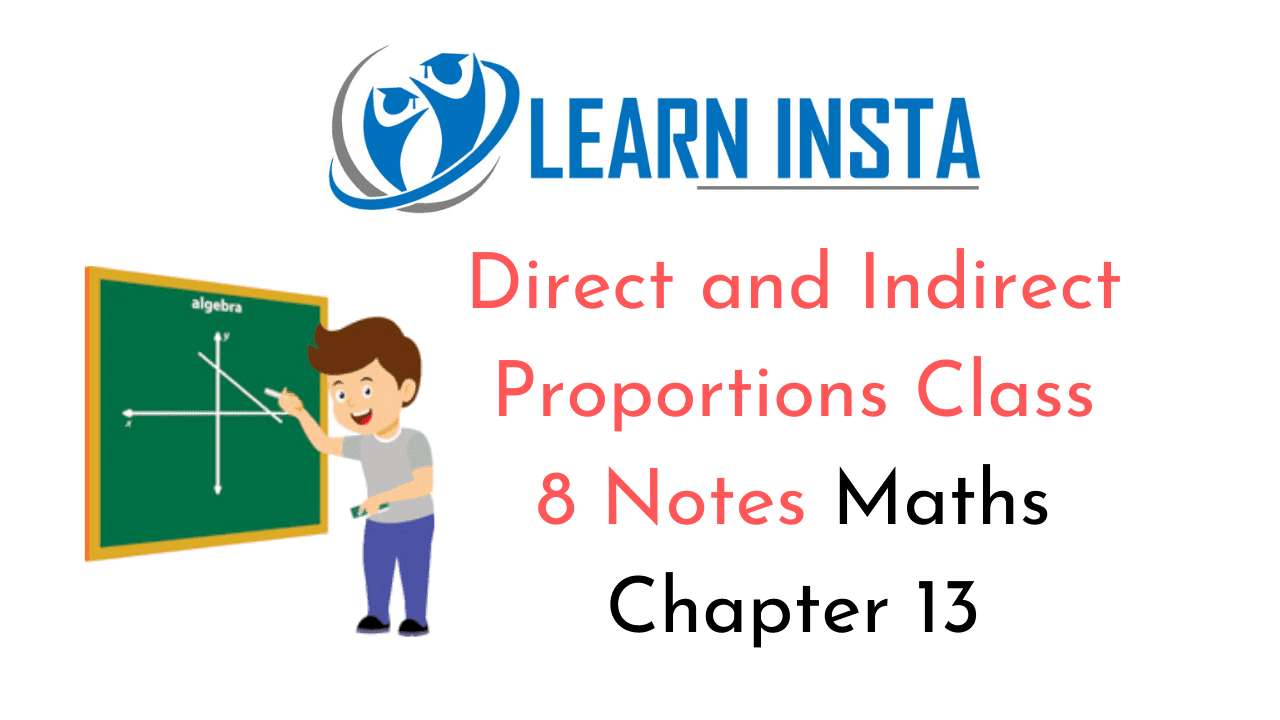On this page, you will find Direct and Indirect Proportions Class 8 Notes Maths Chapter 13 Pdf free download. CBSE NCERT Class 8 Maths Notes Chapter 13 Direct and Indirect Proportions will seemingly help them to revise the important concepts in less time.

## CBSE Class 8 Maths Chapter 13 Notes Direct and Indirect Proportions

### Direct and Indirect Proportions Class 8 Notes Conceptual Facts

1. The two quantities are said to be directly proportional if

• an increase in one leads to a corresponding increase in the other.
• a decrease in one leads to a corresponding decrease in the other.

2. If x varies directly as y then the ratio $$\frac{x}{y}=k$$ (constant) symbolically, x α y, where α is symbol of
proportionality or x = ky $$\frac{x_{1}}{y_{1}}=\frac{x_{2}}{y_{2}}=\frac{x_{3}}{y_{3}}=k$$

3. The two quantities are said to be inversely proportional if

• an increase in one leads to a corresponding decrease in the other.
• a decrease in one leads to a corresponding increase in the other.

4. If x varies inversely with y, then x α $$\frac{1}{y}$$ ⇒ xy = k(constant) or xy – x1y1 = x2y2 = k.

5. Speed =$$\frac{\text { Distance }}{\text { Time }}$$ or Distance = Speed x time or time =$$\frac{\text { Distance }}{\text { Speed }}$$